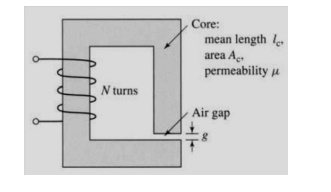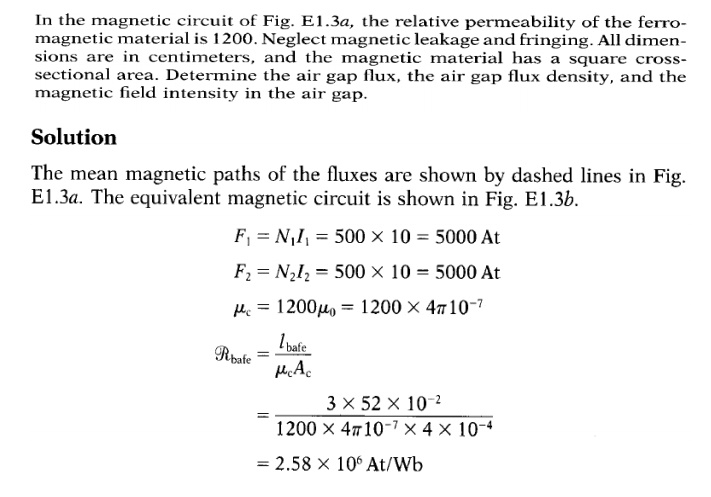Home | | Electrical Machines I | Solved problems - Magnetic Circuits and Magnetic Materials

# Solved problems - Magnetic Circuits and Magnetic Materials

Electrical machines - Magnetic Circuits and Magnetic Materials

Solved problems

Eg .No.1

A magnetic circuit with a single air gap is shown in Fig. 1.24. The core dimensions are:

Cross-sectional area Ac = 1.8 × 10-3 m2

Mean core length lc = 0.6 m

Gap length g = 2.3 x 10-3 m

N = 83 turnsAssume that the core is of infinite permeability ( m -> ¥) and neglect the effects of fringing fields at the air gap and leakage flux. (a) Calculate the reluctance of the core

Re and that of the gap Rg. For a current of i = 1.5 A, calculate (b) the total flux ϕ, (c) the flux linkages λ of the coil, and (d) the coil inductance L.Eg .No.2

Consider the magnetic circuit of with the dimensions of Problem 1.1. Assuming infinite core permeability, calculate (a) the number of turns required to achieve an inductance of 12 mH and (b) the inductor current which will result in a core flux density of 1.0 T.Eg .No.3

A square voltage wave having a fundamental frequency of 60 Hz and equal positive and negative half cycles of amplitude E is applied to a 1000-turn winding surrounding a closed iron core of 1.25 x 10-3m2 cross section. Neglect both the winding resistance and any effects of leakage flux.

(a)                       Sketch the voltage, the winding flux linkage, and the core flux as a function of time.

(b)                       Find the maximum permissible value of E if the maximum flux density is not to exceed 1.15 T.Eg.No.4Study Material, Lecturing Notes, Assignment, Reference, Wiki description explanation, brief detail
Electrical machines : Magnetic Circuits and Magnetic Materials : Solved problems - Magnetic Circuits and Magnetic Materials |